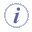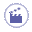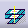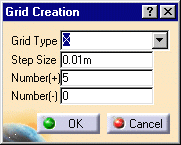# Using Construction PlanesThis task shows you how to create planes to use as references as you construct and position the elements in a drawing.Construction planes are created relative to the axis of the active element, so make sure that the appropriate element (usually an area) is active.1. Select the Construction Planes button. The Grid Creation dialog box is displayed.2. Specify the parameter values for the grid: Construction planes are created outward from the element's axis. If you are creating construction planes in an area along the X or Y axis, as soon as you enter a step size, the system calculates the number of construction planes that will fit in each direction and displays the values in the following fields: If you select an XY Grid Type, the number of steps is calculated, and the Number(+) and Number (-) fields are not displayed. If you are creating construction planes in free space or along the Elevation (Z) axis, the number of steps cannot be calculated. A default value is used for the number of steps. When the Grid Type is set to Elevation (Z), the number of steps is displayed as: You can change the number of steps. 3. Select OK to create the construction planes.To use construction planes for routing or positioning elements, set the Snap Mode to one of the construction plane options on the General Environment Tool Bar.